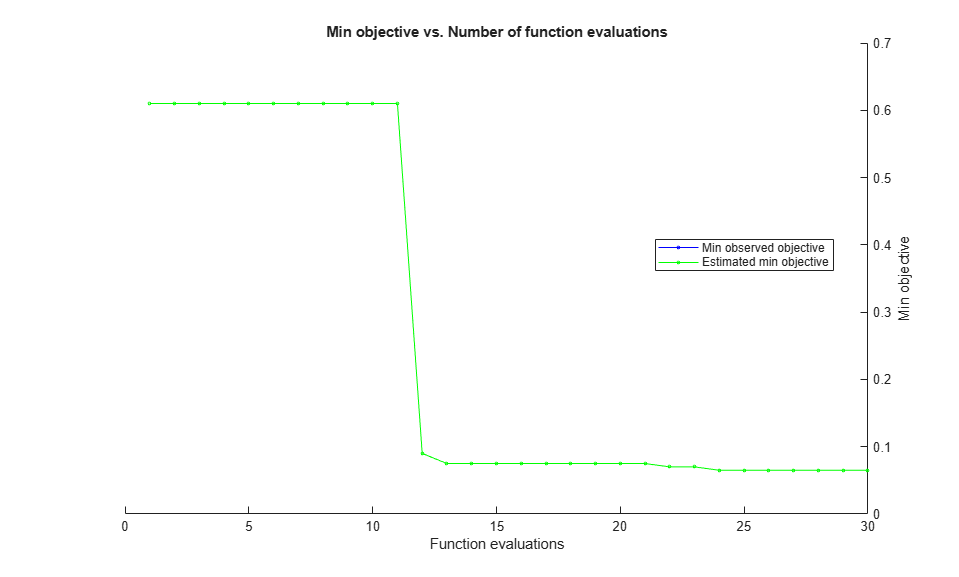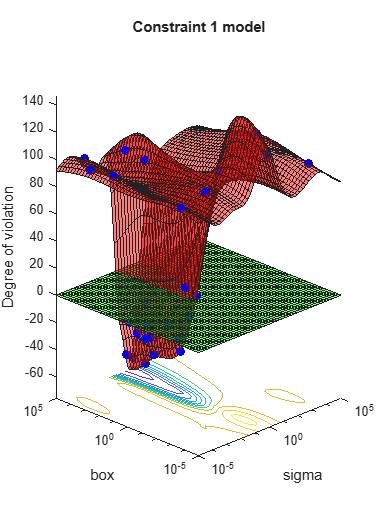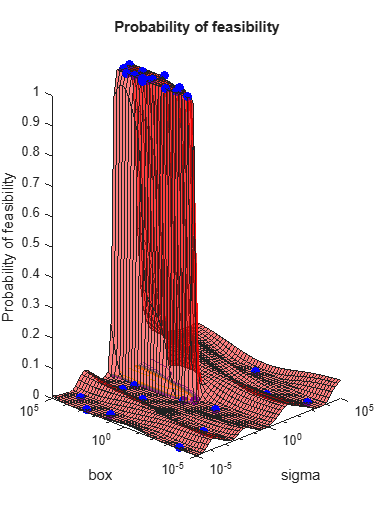Documentation

## Constraints in Bayesian Optimization

### Bounds

`bayesopt` requires finite bounds on all variables. (`categorical` variables are, by nature, bounded in their possible values.) Pass the lower and upper bounds for real and integer-valued variables in `optimizableVariable`.

`bayesopt` uses these bounds to sample points, either uniformly or log-scaled. You set the scaling for sampling in `optimizableVariable`.

For example, to constrain a variable `X1` to values between `1e-6` and `1e3`, scaled logarithmically,

`xvar = optimizableVariable('X1',[1e-6,1e3],'Transform','log')`

`bayesopt` includes the endpoints in its range. Therefore, you cannot use 0 as a lower bound for a log-transformed variable.

### Tip

To use a zero lower bound in a log-transformed variable, set the lower bound to `1`, then inside the objective function use `x-1`.

### Deterministic Constraints — `XConstraintFcn`

Sometimes your problem is valid or well-defined only for points in a certain region, called the feasible region. A deterministic constraint is a deterministic function that returns `true` when a point is feasible, and `false` when a point is infeasible. So deterministic constraints are not stochastic, and they are not functions of a group of points, but of individual points.

### Tip

It is more efficient to use `optimizableVariable` bounds, instead of deterministic constraints, to confine the optimization to a rectangular region.

Write a deterministic constraint function using the signature

`tf = xconstraint(X)`
• `X` is a width-D table of arbitrary height.

• `tf` is a logical column vector, where `tf(i) = true` exactly when `X(i,:)` is feasible.

Pass the deterministic constraint function in the `bayesopt` `XConstraintFcn` name-value pair. For example,

`results = bayesopt(fun,vars,'XConstraintFcn',@xconstraint)`

`bayesopt` evaluates deterministic constraints on thousands of points, and so runs faster when your constraint function is vectorized. See Vectorization (MATLAB).

For example, suppose that the variables named `'x1'` and `'x2'` are feasible when the norm of the vector `[x1 x2]` is less than `6`, and when `x1 <= x2`. The following constraint function evaluates these constraints.

```function tf = xconstraint(X) tf1 = sqrt(X.x1.^2 + X.x2.^2) < 6; tf2 = X.x1 <= X.x2; tf = tf1 & tf2;```

### Conditional Constraints — `ConditionalVariableFcn`

Conditional constraints are functions that enforce one of the following two conditions:

• When some variables have certain values, other variables are set to given values.

• When some variables have certain values, other variables have `NaN` or, for categorical variables, `<undefined>` values.

Specify a conditional constraint by setting the `bayesopt` `ConditionalVariableFcn` name-value pair to a function handle, say `@condvariablefcn`. The `@condvariablefcn` function must have the signature

`Xnew = condvariablefcn(X)`
• `X` is a width-`D` table of arbitrary height.

• `Xnew` is a table the same type and size as `X`.

`condvariablefcn` sets `Xnew` to be equal to `X`, except it also sets the relevant variables in each row of `Xnew` to the correct values for the constraint.

### Note

If you have both conditional constraints and deterministic constraints, `bayesopt` applies the conditional constraints first. Therefore, if your conditional constraint function can set variables to `NaN` or `<undefined>`, ensure that your deterministic constraint function can process these values correctly.

Conditional constraints ensure that variable values are sensible. Therefore, `bayesopt` applies conditional constraints first so that all passed values are sensible.

#### Conditional Constraint That Sets a Variable Value

Suppose that you are optimizing a classification using `fitcdiscr`, and you optimize over both the `'DiscrimType'` and `'Gamma'` name-value pair arguments. When `'DiscrimType'` is one of the quadratic types, `'Gamma'` must be `0` or the solver errors. In that case, use this conditional constraint function:

```function XTable = fitcdiscrCVF(XTable) % Gamma must be 0 if discrim type is a quadratic XTable.Gamma(ismember(XTable.DiscrimType, {'quadratic',... 'diagQuadratic','pseudoQuadratic'})) = 0; end ```

#### Conditional Constraint That Sets a Variable to `NaN`

Suppose that you are optimizing a classification using `fitcsvm`, and you optimize over both the `'KernelFunction'` and `'PolynomialOrder'` name-value pair arguments. When `'KernelFunction'` is not `'polynomial'`, the `'PolynomialOrder'` setting does not apply. The following function enforces this conditional constraint.

```function Xnew = condvariablefcn(X) Xnew = X; Xnew.PolynomialOrder(Xnew.KernelFunction ~= 'polynomial') = NaN;```

You can save a line of code as follows:

```function X = condvariablefcn(X) X.PolynomialOrder(Xnew.KernelFunction ~= 'polynomial') = NaN;```

In addition, define an objective function that does not pass the `'PolynomialOrder'` name-value pair argument to `fitcsvm` when the value of `'PolynomialOrder'` is `NaN`.

```fun = @(X)mysvmfun(X,predictors,responce,c) function objective = mysvmfun(X,predictors,response,c) args = {predictors,response, ... 'CVPartition',c, ... 'KernelFunction',X.KernelFunction}; if ~isnan(X.PolynomialOrder) args = [args,{'PolynomialOrder',X.PolynomialOrder}]; end objective = kfoldLoss(fitcsvm(args{:})); end```

### Coupled Constraints

Coupled constraints are constraints that you can evaluate only by calling the objective function. These constraints can be stochastic or deterministic. Return these constraint values from your objective function in the second argument. See Bayesian Optimization Objective Functions.

The objective function returns a numeric vector for the coupled constraints, one entry for each coupled constraint. For each entry, a negative value indicates that the constraint is satisfied (also called feasible). A positive value indicates that the constraint is not satisfied (infeasible).

`bayesopt` automatically creates a coupled constraint, called the Error constraint, for every run. This constraint enables `bayesopt` to model points that cause errors in objective function evaluation. For details, see Objective Function Errors and `predictError`.

If you have coupled constraints in addition to the Error constraint:

• Include the `NumCoupledConstraints` name-value pair in your `bayesopt` call (required). Do not include the Error constraint in this number.

• If any of your coupled constraints are stochastic, include the `AreCoupledConstraintsDeterministic` name-value pair and pass `false` for any stochastic constraint.

Observe the coupled constraint values in each iteration by setting the `bayesopt` `Verbose` name-value pair to `1` or `2`.

### Note

When there are coupled constraints, iterative display and plot functions can give counterintuitive results such as:

• A minimum objective plot can increase.

• The optimization can declare a problem infeasible even when it showed an earlier feasible point.

The reason for this behavior is that the decision about whether a point is feasible can change as the optimization progresses. `bayesopt` determines feasibility with respect to its constraint model, and this model changes as `bayesopt` evaluates points. So a “minimum objective” plot can increase when the minimal point is later deemed infeasible, and the iterative display can show a feasible point that is later deemed infeasible.

For an example, see Bayesian Optimization with Coupled Constraints.

### Bayesian Optimization with Coupled Constraints

A coupled constraint is one that can be evaluated only by evaluating the objective function. In this case, the objective function is the cross-validated loss of an SVM model. The coupled constraint is that the number of support vectors is no more than 100. The model details are in Optimize a Cross-Validated SVM Classifier Using bayesopt.

Create the data for classification.

```rng default grnpop = mvnrnd([1,0],eye(2),10); redpop = mvnrnd([0,1],eye(2),10); redpts = zeros(100,2); grnpts = redpts; for i = 1:100 grnpts(i,:) = mvnrnd(grnpop(randi(10),:),eye(2)*0.02); redpts(i,:) = mvnrnd(redpop(randi(10),:),eye(2)*0.02); end cdata = [grnpts;redpts]; grp = ones(200,1); grp(101:200) = -1; c = cvpartition(200,'KFold',10); sigma = optimizableVariable('sigma',[1e-5,1e5],'Transform','log'); box = optimizableVariable('box',[1e-5,1e5],'Transform','log'); ```

The objective function is the cross-validation loss of the SVM model for partition `c`. The coupled constraint is the number of support vectors minus 100.5. This ensures that 100 support vectors give a negative constraint value, but 101 support vectors give a positive value. The model has 200 data points, so the coupled constraint values range from -99.5 (there is always at least one support vector) to 99.5. Positive values mean the constraint is not satisfied.

```function [objective,constraint] = mysvmfun(x,cdata,grp,c) SVMModel = fitcsvm(cdata,grp,'KernelFunction','rbf',... 'BoxConstraint',x.box,... 'KernelScale',x.sigma); cvModel = crossval(SVMModel,'CVPartition',c); objective = kfoldLoss(cvModel); constraint = sum(SVMModel.IsSupportVector)-100.5; ```

Pass the partition `c` and fitting data `cdata` and `grp` to the objective function `fun` by creating `fun` as an anonymous function that incorporates this data. See Parameterizing Functions (MATLAB).

```fun = @(x)mysvmfun(x,cdata,grp,c); ```

Set the `NumCoupledConstraints` to `1` so the optimizer knows that there is a coupled constraint. Set options to plot the constraint model.

```results = bayesopt(fun,[sigma,box],'IsObjectiveDeterministic',true,... 'NumCoupledConstraints',1,'PlotFcn',... {@plotMinObjective,@plotConstraintModels},... 'AcquisitionFunctionName','expected-improvement-plus','Verbose',0); ```Most points lead to an infeasible number of support vectors.